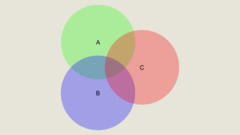## Statistics One

 所在平台: Coursera 课程类别: 统计和数据分析 授课老师： Andrew ConwayExplore 1600+ online courses from top universities. Join Coursera today to learn data science, programming, business strategy, and more.#### 课程详情

Statistics One is designed to be a comprehensive yet friendly introduction to fundamental concepts in statistics. Comprehensive means that this course provides a solid foundation for students planning to pursue more advanced courses in statistics. Friendly means exactly that. The course assumes very little background knowledge in statistics and introduces new concepts with several fun and easy to understand examples.

This course is, quite literally, for everyone. If you think you can't learn statistics, this course is for you. If you had a statistics course before but feel like you need a refresher, this course is for you. Even if you are a relatively advanced researcher or analyst, this course provides a foundation and a context that helps to put one’s work into perspective.

Statistics One also provides an introduction to the R programming language. All the examples and assignments will involve writing code in R and interpreting R output. R software is free! What this means is you can download R, take this course, and start programming in R after just a few lectures. That said, this course is not a comprehensive guide to R or to programming in general.

#### 课程大纲

Lecture Topics

• Lecture 1: Experimental research
• Lecture 2: Correlational research
• Lecture 3: Variables and distributions
• Lecture 4: Summary statistics
• Lecture 5: Correlation
• Lecture 6: Measurement
• Lecture 7: Introduction to regression
• Lecture 8: Null Hypothesis Significance Tests (NHST)
• Lecture 9: Sampling
• Lecture 10: Central limit theorem
• Lecture 11: Multiple regression
• Lecture 12: The General Linear Model (GLM)
• Lecture 13: Moderation
• Lecture 14: Mediation
• Lecture 15: Group comparisons (t-tests)
• Lecture 16: Group comparisons (ANOVA)
• Lecture 17: Factorial ANOVA
• Lecture 18: Repeated measures ANOVA
• Lecture 19: Chi-square
• Lecture 20 Binary logistic regression
• Lecture 21: Assumptions revisited (correlation and regression)
• Lecture 22: Generalized Linear Model
• Lecture 23: Assumptions revisited (t-tests and ANOVA)
• Lecture 24: Non-parametrics (Mann-Whitney U, Kruskal-Wallis)

Lab Topics:
• Lab 2: Histograms, box plots, and descriptives
• Lab 3: Scatterplots and correlation
• Lab 4: Regression
• Lab 5: Confidence intervals
• Lab 6: Multiple regression
• Lab 7: Moderation and mediation
• Lab 8: Group comparisons (t-tests, ANOVA, post-hoc tests)
• Lab 9: Factorial ANOVA
• Lab 10: Chi-square
• Lab 11: Non-linear regression (Binary logistic and Poisson)
• Lab 12: Non-parametrics (Mann-Whitney U and Kruskal-Wallis)

#### 课程评论(4条)

 0 都柏林的老菲利普 2014-01-10 08:56 0 票支持; 0 票反对 不过整体上对于了解一下statistics和相关的应用还是很好的
 0 都柏林的老菲利普 2014-01-10 08:55 0 票支持; 0 票反对 Hypothesis testing那段的评论明显讲的有问题。
 0 申砾 2013-10-20 23:05 0 票支持; 0 票反对 教授讲的很清晰，有不少例子，一些基本概念也会详细讲解，学了之后对R语言也算是入门了。另外课程的forum很活跃，看大家的讨论也收获不少。
 0 宋鑫要学习 2013-10-12 13:41 0 票支持; 0 票反对 刚上了两次课，讲的都是很简单的概念，对想从事机器学习/数据挖掘而又缺乏统计知识的同学是个不错的选择。另外，这门课的lab用的是R语言，对想要入门R的同学也是一个不错的选择